# Evaluation

## Comparison of CFD Calculations with Experiments

For both “weak” and “strong” Mach number cases,for comparison with the calculations, experimental data for four measurements stations after the shock-wave (h1, h2, h3, h4) are selected for the longitudinal velocity as well as pressure distributions along the bottom and the top wall of the diffuser. A representative figure of the shock-wave positions and the measurement stations is shown in fig.9. The x-location of the axial measurement stations are non-dimensionalized with the diffuser throat height,resulting in the following four stations: h1 = 2.822, h2 = 4.611, h3 = 6.340 and h4 = 7.493.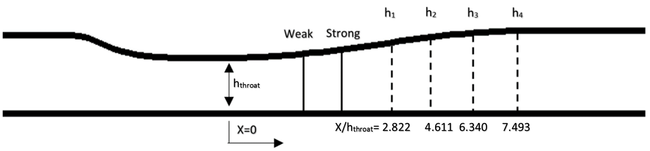Figure 9: Shock-waves positions and experimental measurements

### The weak Mach number case

The modelling results of the three adopted turbulence models are compared with the available experimental data for the "weak" Mach number case in figs.10 and 11.

All three turbulent models are able to capture the maximum velocity value. The main differences are observed in the boundary layer in the wall regions. The EVMs have similar behavior as they underpredict the axial velocity,especially in the top wall region of the diverging part of the diffuser. On the other hand,the RSM overpredicts the axial velocity on both walls providing thinner boundary layers. The pressure coefficient distributions along both walls of the diffuser are plotted in fig.11.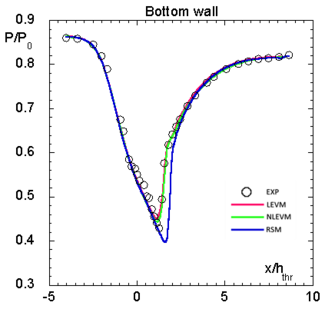|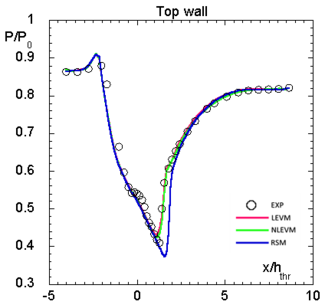Figure 11: Weak shock wave case: Pressure coefficient distributions

All the turbulence models capture the pressure coefficient distribution in the converging and the diverging part of the diffuser with a very good accuracy. However,in the region near the shock-wave (the region where the minimum pressure value is computed), the good level of accuracy is not maintained. The EVMs compute a less intense shock-wave, as it can be concluded from fig.11. Regarding the RSM, it computes a shock-wave with a greater value for the maximum Mach number, giving a lower pressure coefficient value.

The static pressure (Pa) and the Mach number contours for all the turbulence models are presented in figs.12 and 13. The flow field representation is almost the same for all the models with the RSM providing the largest Mach number value in comparison with the EVMs.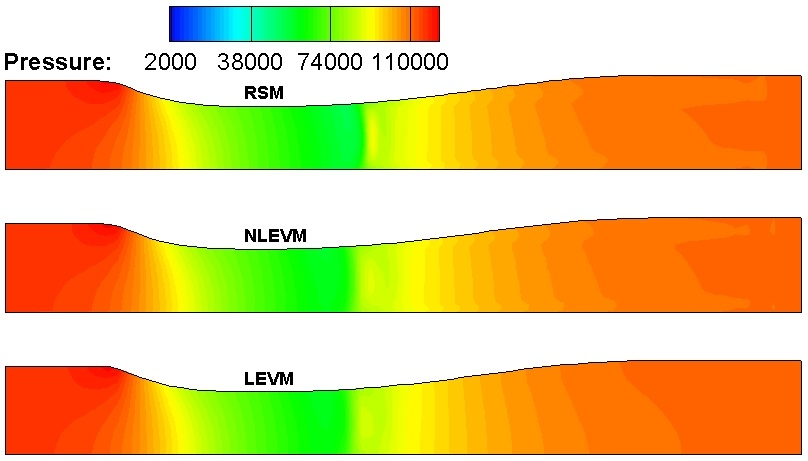Figure 12: Weak shock wave case: Contours of static pressure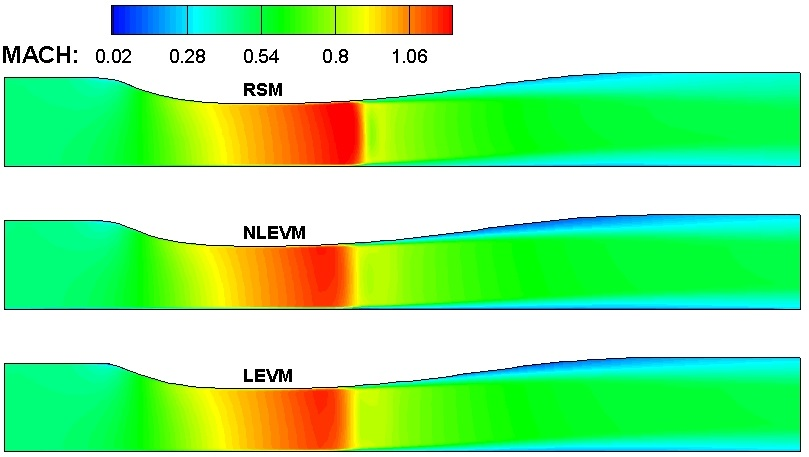Figure 13: Weak shock wave case: Contours of Mach number

### The strong Mach number case

The computed velocity distributions for the EVMs and RSM in comparison with the experimental data are shown in fig.14.

From the first two measurements stations it is evident that the experimental data show a recirculation region formed after the “strong” shock-wave on the top wall of the diverging part of the diffuser. From fig.14 it can be seen that the EVMs are able to capture the boundary layer separation but with a smaller negative velocity resulting in a smaller recirculation region. Additionally,the LEVM computes also a small recirculation zone on the bottom wall, which has an impact on the development of the attached boundary layer further downstream. As a result,the attached boundary layers of the LEVM are thicker in comparison with the computed boundary layers of the other two turbulence models and in relation to the experiment, fig.14. For the first station at x/hthroat = 2.882 only the NLEVM is able to capture the velocity maximum in the core of the flow. However, it gives a lower value of the maximum Mach number as can be concluded from the pressure-coefficient distributions shown in fig.15. Additionally, the NLEVM is the model that presents the greatest accuracy in the calculation of the boundary layer on the bottom wall of the diffuser. Finally, the RSM is not able to capture the flow recirculation on the upper wall, but its results are closer to the experimental data on the bottom wall and in comparison with the LEVM. It must be mentioned that the RSM’s inability to model the boundary layer separation is probably related to the minor modification that has been introduced. Although this modification to the RSM provided numerical stability and results more consistent with the flow physics regarding the intensity of the Mach number, it provided non-physical results regarding the recirculation region since it was not able to predict it as shown in fig.18. Hence, the RSM computation for the “strong” Mach number case with the model in its current form is not appropriate for reaching general conclusions regarding the performance of RSM models for this flow. Further studies on the modelling of the pressure-strain correlation term are needed.

The pressure coefficient distributions for the bottom and the top diverging diffuser wall are presented in fig.15. The NLEVM predicts a higher value of the coefficient and a lower value of the Mach number than was measured. On the other hand, the RSM and the LEVM compute pressure coefficient values slightly lower than the measured ones for the top wall and almost exact for the bottom wall in comparison with the experiments.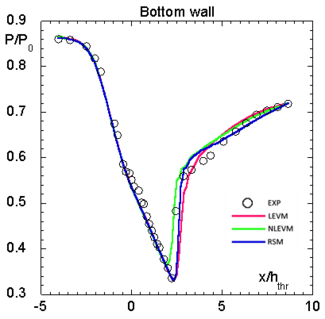|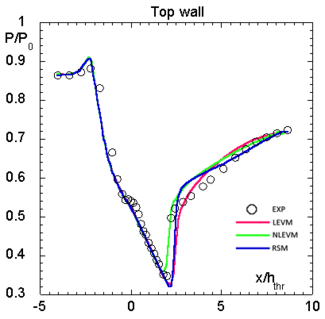Figure 15: Strong shock-wave case: Pressure coefficient distributions

The contours of the static pressure (Pa) distribution and the Mach number for the strong shock-wave case are shown in figs.16 and 17. The NLEVM predicts smaller Mach number values and has a similar behavior as in the weak shock-wave case, in comparison to the other two turbulence models.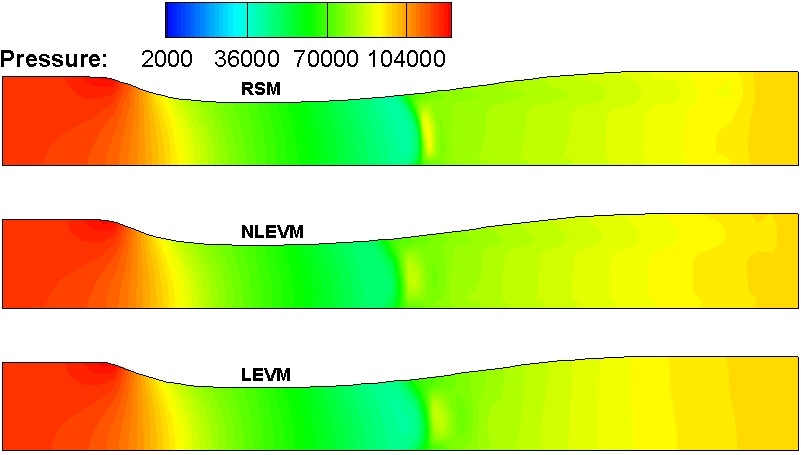Figure 16: Strong shock wave case: Contours of static pressure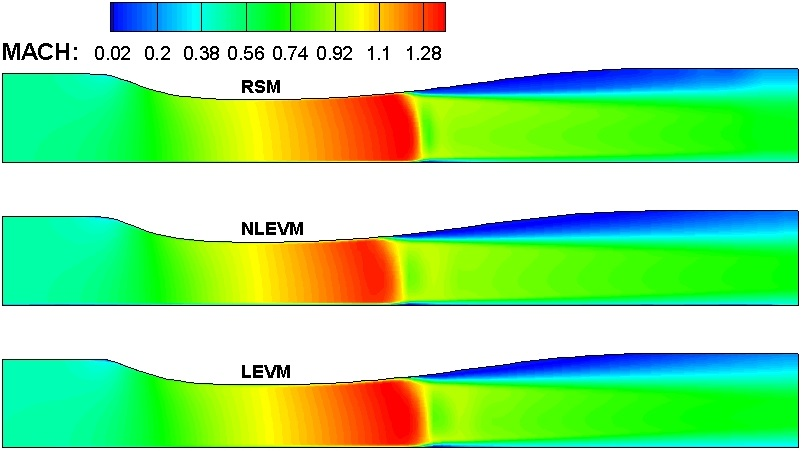Figure 17: Strong shock wave case: Contours of Mach number

Table 4 presents the measured and the modelled separation and reattachment point on the diverging part of the diffuser. Additionally, streamlines focusing on the recirculation region are depicted in fig.18, which also show the separation and reattachment points

Table 4: Separation and reattachment locations on the top wall for the strong shock-wave case
Separation point

(x/hthroat)

Reattachment point

(x/hthroat)

Separation length

(Δx/hthroat)

LEVM 2.49 5.47 2.98
NLEVM 2.13 5.77 3.64
RSM No separation No separation No separation
Experiment 1.98 6 4.02

From table 4 it can be concluded that the EVMs underpredict the total length of the recirculation region. The LEVM computes a smaller recirculation region since the separation point is located further downstream than the measurements show. The NLEVM predictions are much closer to the experiment for the locations of both separation and reattachment points. The RSM, as adopted in the current study, is not able to produce any negative values for the axial velocity and thus computes the flow to be fully attached on the top wall as can be seen from the streamlines in fig.18. As a concluding remark for all the adopted models, the results obtained with the NLEVM for the predicted onset and end of the recirculation region are closer to the experimental data.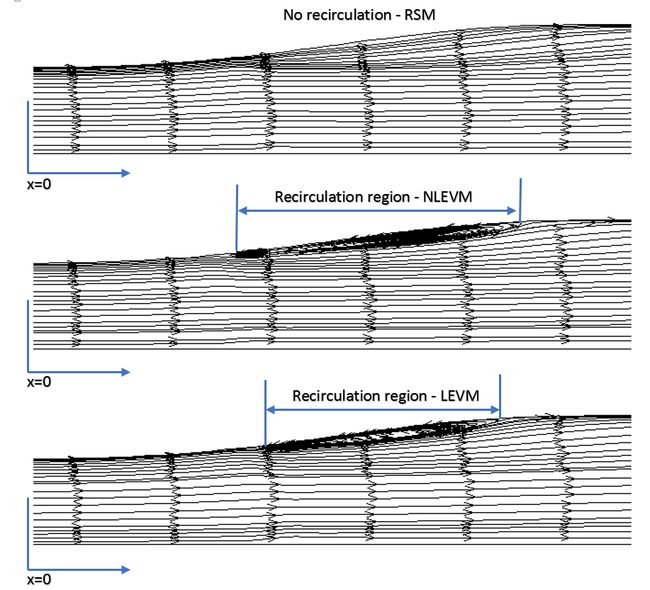Figure 18: Streamlines for the strong Mach number case

### Turbulent kinetic energy and Reynolds-stresses distribution

For the turbulence quantities calculated by the turbulence models, e.g. Reynolds-stresses and turbulent kinetic energy distributions, there are no experimental data available. Hence, in order to assess the more advanced turbulence model behavior regarding the turbulence development in the diffuser transonic flow, a comparison of the normal Reynolds-stress distributions along the same selected locations is presented only for the computed turbulence quantities. The Reynolds-stress values are normalized with the maximum value of the longitudinal stress computed by the RSM, in order to have a qualitative relative comparison since the RSM computes higher longitudinal Reynolds-stress than the EVMs. The normalized normal Reynolds stress distributions i.e. u'u', v'v' and w'w', for the three adopted turbulence models are presented in fig.19 for the “weak” Mach number case. For the “strong” Mach number case they are given in VY. As a general observation, the RSM provides larger values for the normal Reynolds-stresses. Furthermore, the Reynolds-stress anisotropy is clearly better represented by the RSM. The NLEVM can also capture partly the anisotropy (higher values for the longitudinal stress) but yields v'v' ≈ w'w' as discussed further below. Finally, as is well known, LEVM cannot predict the anisotropy as it basically gives a value of 2 / 3k for each normal stress component and should not be used for calculating the individual components.

The higher values of the normal Reynolds-stresses computed by the RSM have a straightforward impact on the calculation the turbulent kinetic energy k which is half the sum of the individual normal stresses. In fig.20, the contours of the turbulent kinetic energy (m2/sec2) are presented for all the turbulence models for the region just after the “weak” shock-wave. From this figure it can be concluded that the RSM predicts the highest values for the turbulent kinetic energy after the shock-wave.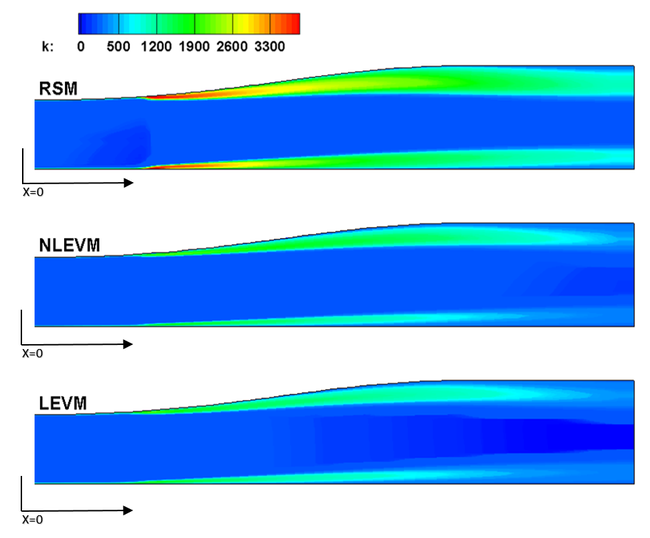Figure 20: Weak shock-wave case: Contours of turbulent kinetic energy

For the “strong” Mach number case, similar observations can be made (see VY). The higher turbulence values generated by the RSM can explain the failure of the RSM to predict the recirculation region. This behavior of the RSM might be linked to the pressure-strain correlation term that was slightly modified in order to obtain more physically consistent results regarding the Mach number distribution.

The smaller values and the lower anisotropy level of the Reynolds-stresses computed by the NLEVM have also been reported by the model originators. The problem is mainly caused by the weak wall damping mechanism of the normal Reynolds-stress component. An improvement of the model was proposed by adding a third transport equation for the stress invariant A2 and by sensitizing the model coefficients with the strain and vorticity invariants, Craft et al. (1997). Regarding the adopted RSM model, it is able to provide the proper anisotropic Reynolds-stress distributions in its present form. However, it has the deficiency that it was not able to provide the proper velocity distributions after the shock-wave for the “strong” Mach number case.

### The effect of dilatation-dissipation

The adopted simple expression of the dilatation-dissipation term has a positive impact on the LEVM computations for the weak shock-wave case, and a negligible one on the other turbulence models computations. The dilatation-dissipation does not alter the quality of the results and seems to have a minor effect especially when used with the more advanced turbulence models. These observations are in agreement with the Morkovin’s hypothesis since the compressibility effects for the Mach number values encountered in the cases studied are accounted for by the mean density variations, as proved by So et al. (1998) who validated the hypothesis with DNS data of a supersonic flat plate boundary layer. The effect of dilatation-dissipation on the computations is shown in table 5.

Table 5: Diffuser maximum Mach number with and without dilatation-dissipation
Weak shock-wave Strong shock-wave
Dilatation-dissipation with without with without
LEVM 1.24 1.08 1.38 1.39
NLEVM 1.24 1.23 1.35 1.36
RSM 1.27 1.28 1.39 1.39
experiment ≈ 1.25 ≈ 1.36

### Diffuser efficiency

Finally, in order to investigate the deviation from the isentropic process, the efficiency of the diffuser, given by the expression

${n_{ef}={\frac {H_{0in}-H_{out}}{H_{0in}-H_{isout}}}}$was calculated. ${H_{0in}}$is the total inlet enthalpy, ${H_{out}}$is the exit enthalpy as computed by the turbulence models and ${H_{isout}}$is the outlet enthalpy of the diffuser that would have been achieved if the process was treated as isentropic. For both the “strong” and the “weak” shock-wave cases, the EVMs calculate efficiency values smaller than the ones computed by the RSM. This was expected since the eddy-viscosity models produce velocity profiles with thicker boundary layers and thus increasing the dissipated energy. Especially for the strong shock-wave case where a recirculation region is captured by the EVMs. The computed efficiencies are presented in table 6.

Table 6: Diffuser efficiency for the weak and strong shock-wave case
Weak shock-wave diffuser efficiency Strong shock-wave diffuser efficiency
LEVM 0.72 0.55
NLEVM 0.75 0.65
RSM 0.87 0.75

Contributed by: Z. Vlahostergios, K. Yakinthos — Dept. of Mechanical Engineering, Aristotle University of Thessaloniki, Greece